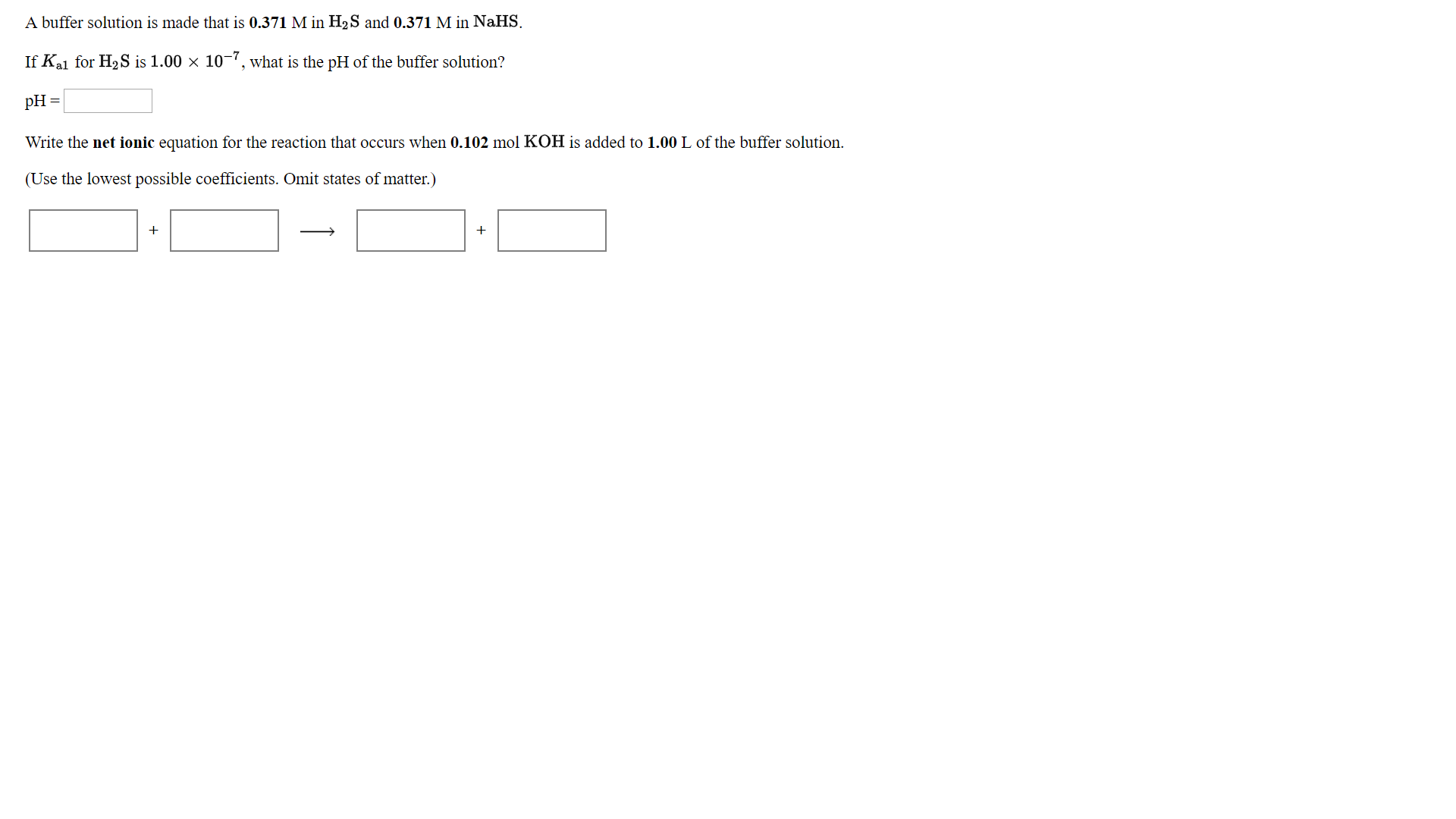# A buffer solution is made that is 0.371 M in H2S and 0.371 M in NaHS.If Kal for H2S is 1.00 x 10-7, what is the pH of the buffer solution?pHWrite the net ionic equation for the reaction that occurs when 0.102 mol KOH is added to 1.00 L of the buffer solution.(Use the lowest possible coefficients. Omit states of matter.)

Question
245 viewshelp_outlineImage TranscriptioncloseA buffer solution is made that is 0.371 M in H2S and 0.371 M in NaHS. If Kal for H2S is 1.00 x 10-7, what is the pH of the buffer solution? pH Write the net ionic equation for the reaction that occurs when 0.102 mol KOH is added to 1.00 L of the buffer solution. (Use the lowest possible coefficients. Omit states of matter.) fullscreen
check_circle

Step 1

The pH of a buffer solution can be calculate...

### Want to see the full answer?

See Solution

#### Want to see this answer and more?

Solutions are written by subject experts who are available 24/7. Questions are typically answered within 1 hour.*

See Solution
*Response times may vary by subject and question.
Tagged in

### Chemistry